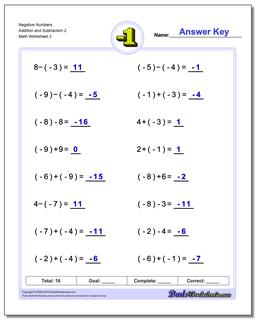# Math Worksheets: Negative Numbers: Negative Numbers: Negative Numbers Addition and Subtraction 2 (Second Worksheet)## Negative Numbers Addition and Subtraction 2 (Second Worksheet)

PropertyValue
DescriptionNegative Numbers Addition and Subtraction 2: Worksheets for adding negative numbers and subtracting negative numbers. (Second Worksheet)
Resource TypeWorksheet